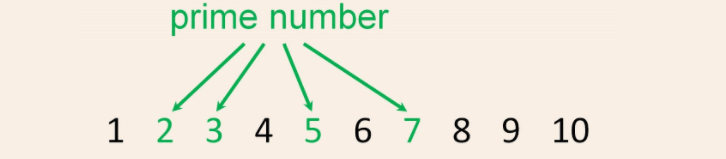Java Tutorial

# Prime Number Program in Java

## What is a Prime Number?

A prime number is defined as a positive integer that possesses precisely two distinct positive integer factors: 1 and itself. In other words, a prime number cannot be expressed as the product of two smaller positive integers. It stands out as a fundamental concept in number theory and plays a crucial role in various mathematical and computational algorithms. Understanding prime numbers is essential in cryptography, where they are utilized for secure data encryption and decryption processes.

Here is a list of all the prime numbers up to 100 :

1. 2,3,5,7,11,13,17,19,23,29,31,37,41,43,47,53,59,61,67,71,73,79,83,89,97

## Prime Number Program in Java

To check whether a number is prime or not in Java, we need to verify if the number has exactly two positive integer factors: 1 and the number itself. If it satisfies this condition, it is a prime number; otherwise, it is not.

Following is a Java program to determine whether a given number is prime or not:

public class PrimeNumberChecker { public static boolean isPrime(int number) { if (number <= 1) { return false; // Numbers less than or equal to 1 are not prime } for (int i = 2; i <= Math.sqrt(number); i++) { if (number % i == 0) { return false; // If the number is divisible by any integer between 2 and its square root, it's not prime } } return true; // If no factors are found, the number is prime } public static void main(String[] args) { int num = 17; // Change this number to check other values if (isPrime(num)) { System.out.println(num + " is a prime number."); } else { System.out.println(num + " is not a prime number."); } } }

In this program, the isPrime method takes an integer number as input and returns true if it's prime and false otherwise. It starts by handling the cases where the input number is less than or equal to 1, as these numbers are not prime. Then, it uses a for loop to check if the number is divisible by any integer from 2 to its square root. If it finds any such factor, it returns false, indicating that the number is not prime. If no factors are found, it returns true, indicating that the number is prime.### Conclusion

In the main method, we call the isPrime method with a sample number (here, num = 17) and display the result accordingly. You can change the value of num to test other numbers for primality.